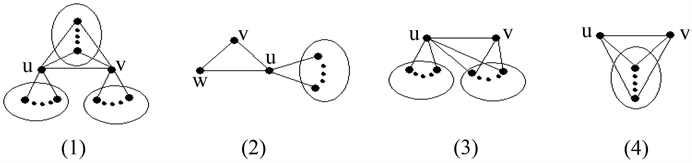# 变换图G---的Wiener指标Wiener Index of Transformation Graph G---

• 全文下载: PDF(619KB)    PP.703-707   DOI: 10.12677/AAM.2019.84080
• 下载量: 269  浏览量: 400

The transformation graph G--- of a graph G is the graph with vertex set V(G)∪E(G), in which two vertices u and v are joined by an edge if one of the following conditions holds: 1) u,v∈V(G) and they are not adjacent in G, 2) u,v∈E(G) and they are not adjacent in G, 3) one of u and v is in V(G) while the other is in E(G), and they are not incident in G. The Wiener index W(G) of G is the sum of the distances between all pairs of vertices in G. In this note, for any graph G, we de-termine the Wiener index of G---, when G--- is connected.

1. 引言

2. 主要内容

2.1. 预备知识

2.2. 直径小于等于2Figure 1. $\text{diam}\left({G}^{---}\right)=3$

$W\left({G}^{---}\right)=\frac{1}{2}\left({m}^{2}+{n}^{2}+\underset{v\in V\left(G\right)}{\sum }{d}_{G}^{2}\left(v\right)\right)+mn+\frac{3}{2}m-\frac{1}{2}n.$

$\begin{array}{c}W\left({G}^{---}\right)=\underset{u,v}{\sum }{d}_{{G}^{---}}\left(u,v\right)+\underset{u,e}{\sum }{d}_{{G}^{---}}\left(u,e\right)+\underset{e,f}{\sum }{d}_{{G}^{---}}\left(e,f\right)\\ =\left(\underset{uv\notin E\left(G\right)}{\sum }{d}_{{G}^{---}}\left(u,v\right)+\underset{uv\in E\left(G\right)}{\sum }{d}_{{G}^{---}}\left(u,v\right)\right)+\left(\underset{{m}_{ue}=0}{\sum }{d}_{{G}^{---}}\left(u,e\right)+\underset{{m}_{ue}=1}{\sum }{d}_{{G}^{---}}\left(u,e\right)\right)\\ +\left(\underset{{m}_{ef}=0}{\sum }{d}_{{G}^{---}}\left(e,f\right)+\underset{{m}_{ef}=1}{\sum }{d}_{{G}^{---}}\left(e,f\right)\right)\\ =\left(\left(\begin{array}{c}n\\ 2\end{array}\right)-m+2m\right)+\left(mn-2m+4m\right)+\left(\left(\begin{array}{c}m\\ 2\end{array}\right)-\underset{v\in V\left(G\right)}{\sum }\left(\begin{array}{c}{d}_{G}\left(v\right)\\ 2\end{array}\right)+2\underset{v\in V\left(G\right)}{\sum }\left(\begin{array}{c}{d}_{G}\left(v\right)\\ 2\end{array}\right)\right)\\ =\frac{1}{2}\left({m}^{2}+{n}^{2}+\underset{v\in V\left(G\right)}{\sum }{d}_{G}^{2}\left(v\right)\right)+mn+\frac{3}{2}m-\frac{1}{2}n.\end{array}$

2.3. 直径等于3

1) $W\left({G}^{---}\right)=\frac{1}{2}\left({m}^{2}+{n}^{2}+\underset{v\in V\left(G\right)}{\sum }{d}_{G}^{2}\left(v\right)\right)+mn+\frac{3}{2}m-\frac{1}{2}n+1$

2) $W\left({G}^{---}\right)=\frac{1}{2}\left({m}^{2}+{n}^{2}+\underset{v\in V\left(G\right)}{\sum }{d}_{G}^{2}\left(v\right)\right)+mn+\frac{3}{2}m-\frac{1}{2}n+4$

3) $W\left({G}^{---}\right)=\frac{1}{2}\left({m}^{2}+{n}^{2}+\underset{v\in V\left(G\right)}{\sum }{d}_{G}^{2}\left(v\right)\right)+mn+\frac{3}{2}m-\frac{1}{2}n+2$

4) $W\left({G}^{---}\right)=\frac{1}{2}\left({m}^{2}+{n}^{2}+\underset{v\in V\left(G\right)}{\sum }{d}_{G}^{2}\left(v\right)\right)+mn+\frac{3}{2}m-\frac{1}{2}n+3$

$\begin{array}{c}W\left({G}^{---}\right)=\underset{u,v}{\sum }{d}_{{G}^{---}}\left(u,v\right)+\underset{u,e}{\sum }{d}_{{G}^{---}}\left(u,e\right)+\underset{e,f}{\sum }{d}_{{G}^{---}}\left(e,f\right)\\ =\left(\underset{uv\notin E\left(G\right)}{\sum }{d}_{{G}^{---}}\left(u,v\right)+\underset{uv\in E\left(G\right)}{\sum }{d}_{{G}^{---}}\left(u,v\right)\right)+\left(\underset{{m}_{ue}=0}{\sum }{d}_{{G}^{---}}\left(u,e\right)+\underset{{m}_{ue}=1}{\sum }{d}_{{G}^{---}}\left(u,e\right)\right)\\ +\left(\underset{{m}_{ef}=0}{\sum }{d}_{{G}^{---}}\left(e,f\right)+\underset{{m}_{ef}=1}{\sum }{d}_{{G}^{---}}\left(e,f\right)\right)\\ =\left(\left(\begin{array}{c}n\\ 2\end{array}\right)-m+2\left(m-1\right)+3\right)+\left(mn-2m+4m\right)+\left(\left(\begin{array}{c}m\\ 2\end{array}\right)-\underset{v\in V\left(G\right)}{\sum }\left(\begin{array}{c}{d}_{G}\left(v\right)\\ 2\end{array}\right)+2\underset{v\in V\left(G\right)}{\sum }\left(\begin{array}{c}{d}_{G}\left(v\right)\\ 2\end{array}\right)\right)\\ =\frac{1}{2}\left({m}^{2}+{n}^{2}+\underset{v\in V\left(G\right)}{\sum }{d}_{G}^{2}\left(v\right)\right)+mn+\frac{3}{2}m-\frac{1}{2}n+1.\end{array}$

$\begin{array}{c}W\left({G}^{---}\right)=\underset{u,v}{\sum }{d}_{{G}^{---}}\left(u,v\right)+\underset{u,e}{\sum }{d}_{{G}^{---}}\left(u,e\right)+\underset{e,f}{\sum }{d}_{{G}^{---}}\left(e,f\right)=\left(\underset{uv\notin E\left(G\right)}{\sum }{d}_{{G}^{---}}\left(u,v\right)+\underset{uv\in E\left(G\right)}{\sum }{d}_{{G}^{---}}\left(u,v\right)\right)\\ +\left(\underset{{m}_{ue}=0}{\sum }{d}_{{G}^{---}}\left(u,e\right)+\underset{{m}_{ue}=1}{\sum }{d}_{{G}^{---}}\left(u,e\right)\right)+\left(\underset{{m}_{ef}=0}{\sum }{d}_{{G}^{---}}\left(e,f\right)+\underset{{m}_{ef}=1}{\sum }{d}_{{G}^{---}}\left(e,f\right)\right)\\ =\left(\left(\begin{array}{c}n\\ 2\end{array}\right)-m+2\left(m-2\right)+3×2\right)+\left(mn-2m+2\left(2m-2\right)+3×2\right)\\ +\left(\left(\begin{array}{c}m\\ 2\end{array}\right)-\underset{v\in V\left(G\right)}{\sum }\left(\begin{array}{c}{d}_{G}\left(v\right)\\ 2\end{array}\right)+2\underset{v\in V\left(G\right)}{\sum }\left(\begin{array}{c}{d}_{G}\left(v\right)\\ 2\end{array}\right)\right)\\ =\frac{1}{2}\left({m}^{2}+{n}^{2}+\underset{v\in V\left(G\right)}{\sum }{d}_{G}^{2}\left(v\right)\right)+mn+\frac{3}{2}m-\frac{1}{2}n+4.\end{array}$

$\begin{array}{c}W\left({G}^{---}\right)=\underset{u,v}{\sum }{d}_{{G}^{---}}\left(u,v\right)+\underset{u,e}{\sum }{d}_{{G}^{---}}\left(u,e\right)+\underset{e,f}{\sum }{d}_{{G}^{---}}\left(e,f\right)\\ =\left(\underset{uv\notin E\left(G\right)}{\sum }{d}_{{G}^{---}}\left(u,v\right)+\underset{uv\in E\left(G\right)}{\sum }{d}_{{G}^{---}}\left(u,v\right)\right)\\ +\left(\underset{{m}_{ue}=0}{\sum }{d}_{{G}^{---}}\left(u,e\right)+\underset{{m}_{ue}=1}{\sum }{d}_{{G}^{---}}\left(u,e\right)\right)+\left(\underset{{m}_{ef}=0}{\sum }{d}_{{G}^{---}}\left(e,f\right)+\underset{{m}_{ef}=1}{\sum }{d}_{{G}^{---}}\left(e,f\right)\right)\\ =\left(\left(\begin{array}{c}n\\ 2\end{array}\right)-m+2\left(m-1\right)+3\right)+\left(mn-2m+2\left(2m-1\right)+3\right)+\left(\left(\begin{array}{c}m\\ 2\end{array}\right)-\underset{v\in V\left(G\right)}{\sum }\left(\begin{array}{c}{d}_{G}\left(v\right)\\ 2\end{array}\right)+2\underset{v\in V\left(G\right)}{\sum }\left(\begin{array}{c}{d}_{G}\left(v\right)\\ 2\end{array}\right)\right)\\ =\frac{1}{2}\left({m}^{2}+{n}^{2}+\underset{v\in V\left(G\right)}{\sum }{d}_{G}^{2}\left(v\right)\right)+mn+\frac{3}{2}m-\frac{1}{2}n+2.\end{array}$

$\begin{array}{c}W\left({G}^{---}\right)=\underset{u,v}{\sum }{d}_{{G}^{---}}\left(u,v\right)+\underset{u,e}{\sum }{d}_{{G}^{---}}\left(u,e\right)+\underset{e,f}{\sum }{d}_{{G}^{---}}\left(e,f\right)\\ =\left(\underset{uv\notin E\left(G\right)}{\sum }{d}_{{G}^{---}}\left(u,v\right)+\underset{uv\in E\left(G\right)}{\sum }{d}_{{G}^{---}}\left(u,v\right)\right)+\left(\underset{{m}_{ue}=0}{\sum }{d}_{{G}^{---}}\left(u,e\right)+\underset{{m}_{ue}=1}{\sum }{d}_{{G}^{---}}\left(u,e\right)\right)\\ +\left(\underset{{m}_{ef}=0}{\sum }{d}_{{G}^{---}}\left(e,f\right)+\underset{{m}_{ef}=1}{\sum }{d}_{{G}^{---}}\left(e,f\right)\right)\\ =\left(\left(\begin{array}{c}n\\ 2\end{array}\right)-m+2\left(m-1\right)+3\right)+\left(mn-2m+2\left(2m-2\right)+3×2\right)+\left(\left(\begin{array}{c}m\\ 2\end{array}\right)-\underset{v\in V\left(G\right)}{\sum }\left(\begin{array}{c}{d}_{G}\left(v\right)\\ 2\end{array}\right)+2\underset{v\in V\left(G\right)}{\sum }\left(\begin{array}{c}{d}_{G}\left(v\right)\\ 2\end{array}\right)\right)\\ =\frac{1}{2}\left({m}^{2}+{n}^{2}+\underset{v\in V\left(G\right)}{\sum }{d}_{G}^{2}\left(v\right)\right)+mn+\frac{3}{2}m-\frac{1}{2}n+3.\end{array}$

  Bondy, J.A. and Murty, U.S.R. (1976) Graph Theory with Applications. American Elsevier, New York, Macmillan, London.  Wiener, H. (1947) Structural Determination of Paraffin Boiling Point. Journal of the American Chemical Society, 69, 17-20. https://doi.org/10.1021/ja01193a005  Entringer, R.C., Jackson, D.E. and Snyder, D.A. (1976) Distance in Graphs. Czechoslovak Mathematical Journal, 26, 283-296.  Dobrynin, A., Entringer, R. and Gutman, I. (2001) Wiener Index of Trees: Theory and Applications. Acta Applicandae Mathematica, 66, 211-249. https://doi.org/10.1023/A:1010767517079  Wu, B. and Meng, J. (2001) Basic Properties of Total Transformation Graphs. Journal of Mathematical Study, 34, 109-116.  Wu, B., Zhang, L. and Zhang, Z. (2005) The Transformation Graph Gxyz When xyz = −++. Discrete Mathematics, 296, 263-270.  Chen, J. (2006) Super Edge-Connectivity of Two Classes of Transformation Graphs. Doctoral Thesis, Xinjiang University, Urumchi.  Xu, L. and Wu, B. (2008) Transformation Graph . Discrete Mathematics, 308, 5144-5148.